﻿ 基于线纹尺的视觉检测标定方法 The Calibrating Method of Linear-Structural Visual System Based on Line Scale Device

Computer Science and Application
Vol.08 No.08(2018), Article ID:26597,7 pages
10.12677/CSA.2018.88133

The Calibrating Method of Linear-Structural Visual System Based on Line Scale Device

Yingsong Chen, Zhouping Cui, Huijie Zhang, Feng Zeng

Chengdu Technological University, Chengdu Sichuan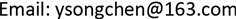Received: Aug. 5th, 2018; accepted: Aug. 20th, 2018; published: Aug. 27th, 2018ABSTRACT

The paper designs a calibrating measurement method based on linear-structural visual sensor of line scale device. The paper proposes a calculation method, which combines line scale planar reference and special points. Through special graphical points in three-dimensional coordinates, we establish subpixel-image index table to directly calibrate the system. The calibrating measurement method is feasible in terms of operating the system, and the calculation process is more convenient. The results of the experiment also prove the effectiveness of this method.

Keywords:Visual Sensor, Line Scale Device, Calibration Method, Linear-Structural Light1. 引言

2. 线纹式靶标的标定

2.1. 直接标定法原理

$\frac{{H}_{1}B}{{M}_{1}D}=\frac{BQ}{DQ}$ (1)

$\frac{y\mathrm{sin}\alpha }{x\mathrm{sin}\beta }=\frac{a-y\mathrm{cos}\alpha }{b+x\mathrm{cos}\beta }$ (2)

$y=\frac{ax\mathrm{sin}\beta }{b\mathrm{sin}\alpha +x\mathrm{sin}\left(\alpha +\beta \right)}$ (3)

y为被测物体表面高度的变化，x是像点M1和M2之间的相移，a是H0点的成像物距，b是H1点的成像相距。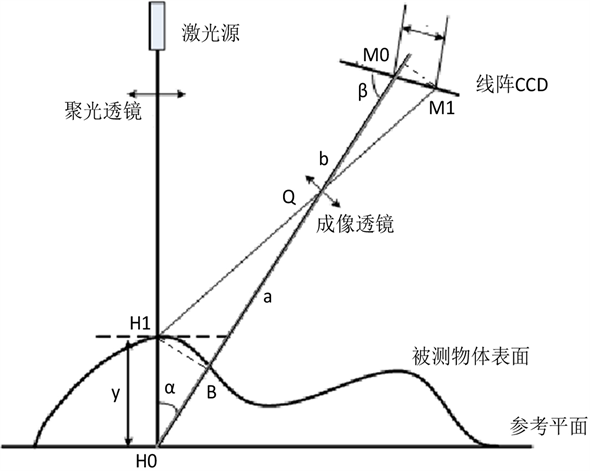Figure 1. Measurement principle of laser triangulation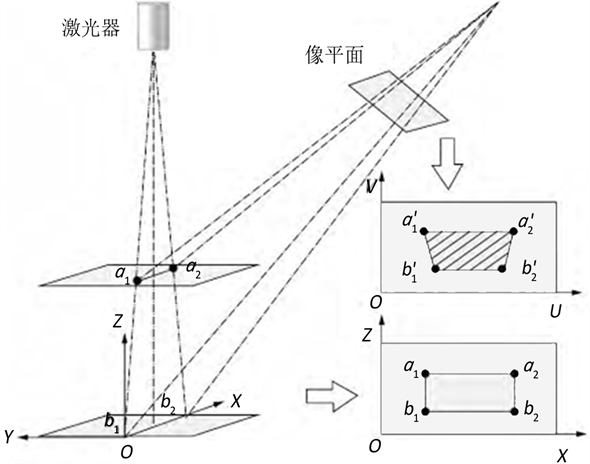Figure 2. Principle of direct calibration

2.2. 线纹靶标特征点的提取

1、提取光条中心

${C}_{j}=\frac{\sum _{i={M}_{j-2}}^{{M}_{j+2}}\left[igray\left(i,j\right)\right]}{\sum _{i={M}_{j-2}}^{{M}_{j+2}}gray\left(i,j\right)}$ (4)

2、提取特征点图像坐标

3、确定特征点三维空间坐标。

${x}_{n}=\left(n-1\right)d$ (5)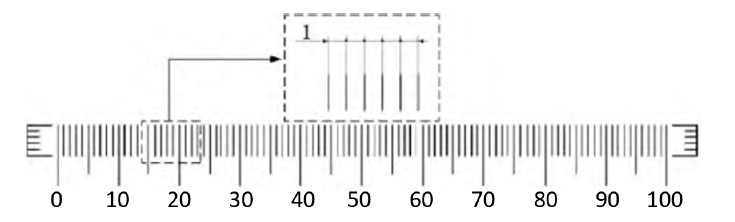Figure 3. Planar target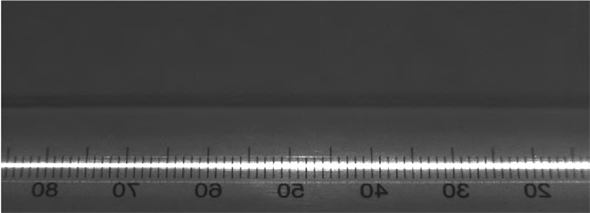(a) 有光条图像(b) 无光条图像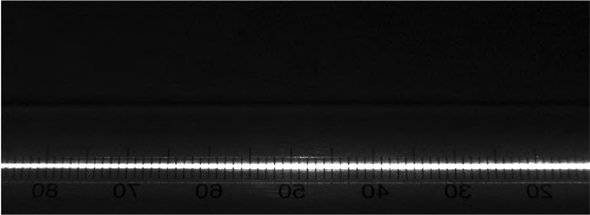(c) 提取光条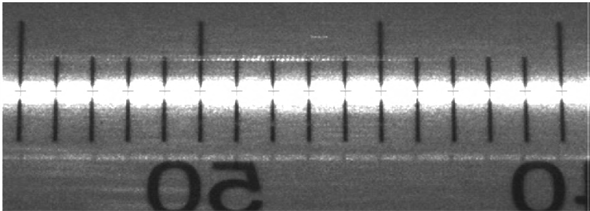(d) 提取交点

Figure 4. Extraction of feature points

3. 标定过程及结果

3.1. 标定过程

1、将线结构光视觉传感器安装在线纹尺自动检定平台上，将基于平面靶标制作成的线纹尺固定放置在位移平台的工作台上。开启线结构光视觉传感器，将线结构光投射到线纹尺上产生如图4(a)的光条，为保证光条在线纹尺两端的刻线上的读数一致并保证光条垂直于线纹尺上的平行线，可以调整线纹尺的位置。

2、采集三维空间坐标系XYZ平面上光平面内不同高度的靶标图像，分以下两步：

1) 确定Z轴的0基准面，调整位移平台Z轴的初始位置，使光条落在目标图像索引表的起始位置。

2) 以0.05 mm的步进距离操作位移平台以改变Z轴的位置，并在同一位置上，获取有光条投射和无光条投射两幅靶标图像。

3、提取靶标图像的特征点，得到各个Z轴上的特征点的三维空间X轴坐标和图像坐标值(U,V)坐标值。

4、建立一个二维数组I，确立的图像坐标值(U,V)与三维空间坐标值(X,Z)之间的对应关系。由于Z轴步进距离和相邻平行线间距的限制，相邻特征点元素在二维数组I中可能存在无赋值的元素，使用插值运算进行赋值，本文采用的是基于三角形的三次插值法。

3.2. 实验结果

1、对Z轴高度的精度分析：将线结构光视觉传感器已标定的工作区域分成8个区域，由于量块材料的高反光性，会在实验过程中会产生光斑，测量区域没有使用中间区域。测量时用1.0000 mm的量块放置于工作台划分的区域上，测量高度为量块中心平面与工作台之间的高度差，重复测量多次，实验结果如表1所示。

2、对Z轴宽度的精度分析：在上述标定的工作区域，实验测量点为光条与平行线的交点，重复测量多次，部分实验结果如表2所示。

4. 结束语

Table1. Measurement data in Z axis

The Calibrating Method of Linear-Structural Visual System Based on Line Scale Device[J]. 计算机科学与应用, 2018, 08(08): 1217-1223. https://doi.org/10.12677/CSA.2018.88133

1. 1. 邝泳聪, 崔亮纯. 基于线纹尺的线结构光视觉传感器标定新方法[J]. 华南理工大学学报: 自然科学版, 2016, 44(1): 71-77.

2. 2. 徐德, 王麟琨, 谭民. 基于运动的手眼系统结构光参数标定[J]. 仪器仪表学报, 2005, 26(11): 1101-1106.

3. 3. 陈应松, 肖世德, 王文玺, 林静. 自主导航移动机器人视觉系统的一种标定方法[J]. 计算机工程与应用, 2009, 45(23): 190-192.

4. 4. 胥磊. 机器视觉技术的发展现状与展望[J]. 设各管理与维修, 2016(9): 7-9.

5. 5. 李炳银. 机器视觉及其在制造业中的应用分析[J]. 数字通信世界, 2017(9): 95.

6. 6. 王运哲, 白雁兵, 张博. 机器视觉系统的设计方法[J]. 现代显示, 2011, 22(11): 24-27.

7. 7. 叶青松. 基于机器视觉的工业检测研究[D]: [硕士学位论文]. 无锡: 江南大学, 2008.

8. 8. 董迪. 基于机器视觉的高精度测量系统研究[D]: [硕士学位论文]. 沈阳: 沈阳工业大学, 2016

9. 9. 聂振宇. 金属部件表面缺陷视觉检测系统研究[D]: [硕士学位论文]. 长沙: 中南大学, 2013.

10. 10. 贾波, 苏湿渝. 采用激光光刀的叶片三维面形测量方法[J]. 中国激光. 1992, 19(4): 271-275.